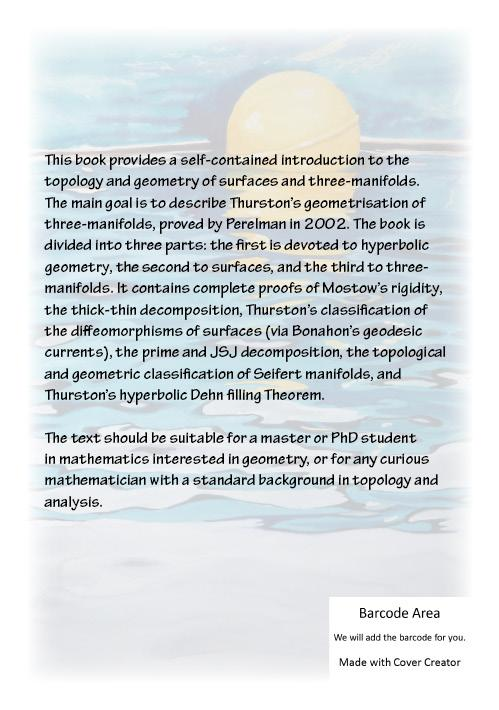An Introduction to Geometric Topology

 I have written an introductory book to Geometric Topology. Here it is: An Introduction to Geometric Topology, CreateSpace Independent Publishing Platform, 488 pages (2016). The book contains 488 pages and 200 colour figures. A printed version (with greyscale figures) can be purchased from Amazon.com or its variants .it, .fr, .uk, .de, .es, .jp ... Copyright license: The book is distributed under the Creative Commons-BY-NC-SA license. This means that you are allowed to distribute, modify, adapt, and use this work for non-commercial purposes, as long as you correctly attribute its authorship and keep using the same license. Table of contents: Part I. Hyperbolic geometry Preliminaries Hyperbolic space Hyperbolic manifolds Thick-thin decomposition The sphere at infinity Part II. Surfaces Surfaces Teichmueller space Surface diffeomorphisms Part III. Three-manifolds Topology of three-manifolds Seifert manifolds Constructions of three-manifolds The eight geometries Mostow rigidity theorem Hyperbolic three-manifolds Hyperbolic Dehn filling Introduction: The aim of this book is to introduce the reader to an area of mathematics called geometric topology. The text should be suitable to a master or PhD student in mathematics interested in geometry, and more generally to any curious mathematician with a standard background in topology and analysis. We interpret here the term "geometric topology" in a quite restrictive sense: for us, this topic is the study of topological manifolds via the assignment of a preferred "geometric structure," that is some Riemannian metric of a particular nice kind. It is one of the most important discoveries in modern geometry that such preferred metrics exist on every compact manifold of dimension two and three, and this is exactly what this book is about. In other words, this book is an introduction to surfaces and three-manifolds, and to their geometrisation, due to Poincare' and Koebe in 1907 in dimension two and to Thurston and Perelmann in 2002 in dimension three. Therefore this is also a textbook on low-dimensional topology, except that we completely neglect four-manifolds, that form a relevant part of this area but which do not (yet?) fit in any geometrisation perspective. There are already many good textbooks on surfaces, so our main new contribution is probably to furnish a complete introduction to Thurston's geometrisation of three-manifolds, that includes both the topological side of the story (the decomposition of three-manifolds along spheres and tori, the classification of Seifert manifolds) and the geometric side (hyperbolic geometry, Thurston's Dehn filling Theorem, Mostow rigidity, the eight geometries). This book is essentially self-contained: in the first chapter we recall all the relevant background material in differential topology and geometry, and from the second chapter on we never mention a theorem without furnishing a proof - with only one important but unavoidable exception: Perelman's solution of the geometrisation conjecture.Back to the main page